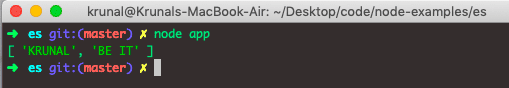# JavaScript Object.values: How to Get Object Values

1
271Before ES2017, to access the JavaScript Object properties, we used the combination of for…in loop and Object.hasOwnProperty() method to access values of own enumerable properties of an object.

But after ES2017, it is no longer needed. Instead, the JavaScript community comes with a function called Object.values() that can help you access the values of enumerable properties of the object.

## JavaScript Object.values

The Object.values() is a built-in JavaScript function that returns the array of the given Object’s enumerable property values. It an object as an argument of which enumerable own property values are to be returned and return the array containing all the enumerable property values of the given Object.

The ordering of the properties is the same as that given by the Object manually if the loop is applied to the properties.

### Syntax

`Object.values(obj)`

### Parameters

An obj parameter whose enumerable own property values are to be returned.

### Return Value

The values() function returns an array containing the given Object’s enumerable property values.

### Example

To run the Object.values(), I am using Node.js, which can run the Javascript programs. Create an app.js file, add the following code, go to your terminal, go inside this filepath and type the node app.js command. It will run the file and give you an output.

```// app.js

let student = { name: 'KRUNAL', education: 'BE IT' };
console.log(Object.values(student));```

The output is the following.So, it has returned an array of values of the passed Object.

We can do the same thing using the for…in loop.

See the following example.

```// app.js

let student = { name: 'KRUNAL', education: 'BE IT' };
let arr = [];
for (const prop in student) {
arr.push(student[prop]);
}
console.log(arr);```

The output is the following.But, we do not have to use for…in loop whenever we have a ready-made method available to get the values of an object in the form of an array. So, for example, you can get an array of keys with Object.keys() and then use the Javascript map() method to get values.

See the following example.

```// app.js

let student = { name: 'KRUNAL', education: 'BE IT' };
let output = Object.keys(student).map(function(e) {
return student[e]
})
console.log(output);```

It will give us the same output.

## Accessing object values using for…in loop

We can access the values of the Object using for…in loop.

See the following code example.

```// app.js

let data = {
name: 'Eleven',
show: 'Stranger Things',
portrayedBy: 'Millie Bobby Brown'
}

for (key in data) {
if (data.hasOwnProperty(key)) {
console.log(data[key])
}
}
```

See the following output.

```➜  es git:(master) ✗ node app
Eleven
Stranger Things
Millie Bobby Brown
➜  es git:(master) ✗
```

## Converting object values to string

To convert object values to string in JavaScript, use the JSON.stringify() method.

See the following code.

```let obj = { name: "Millie", age: 15, city: "London" };

console.log(JSON.stringify(obj))```

See the output.

```➜  es git:(master) ✗ node app
{"name":"Millie","age":15,"city":"London"}
➜  es git:(master) ✗```

## Mapping of Object values in JavaScript

There is no native map to the Object, but you can map the values using the following method.

Let’s append the string to all the object values.

```let obj = { name: "Millie", age: 15, city: "London" };

Object.keys(obj).map(function(key, index) {
obj[key] += 'App';
});

console.log(obj);```

See the output.

```➜  es git:(master) ✗ node app
{ name: 'MillieApp', age: '15App', city: 'LondonApp' }
➜  es git:(master) ✗```

We have appended the string ‘App‘ to all the Object values in the above code.

If you want to get the array of values using the map() function, then use the following code.

```// app.js

let obj = { name: "Millie", age: 15, city: "London" };

data = Object.keys(obj).map(function (key) { return obj[key] })
console.log(data)```

#### Output

```node app
[ 'Millie', 15, 'London' ]```

## Creating a custom Obj.values() function

Let’s say your browser does not support the Object.values() function, and then you can create your basic prototype function using the following code.

```Object.values = function (obj) {
var vals = [];
for( var key in obj ) {
if ( obj.hasOwnProperty(key) ) {
vals.push(obj[key]);
}
}
return vals;
}```

Now, you can call the values function and pass the Object that will return the array of object values.

Depending on which browsers you need to support, we can still use the. Object.values() function.

The majority of browsers in the wild support ECMAScript 5 (ES5), but be warned that many of the examples use Object.keys(), which is not available in IE < 9.

You can see the compatibility table.

That’s it for this example.

## Recommended Posts

Javascript Object toString()

Javascript Object defineProperty()

Javascript Object freeze()

Javascript Object is()

Javascript Object create()

Javascript Object assign()

#### 1 Comment

1.Azmi